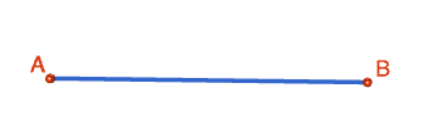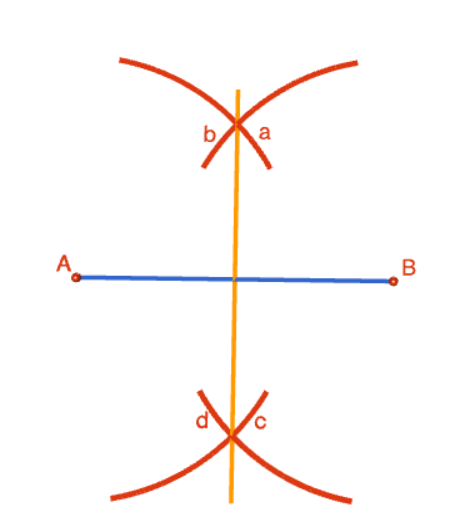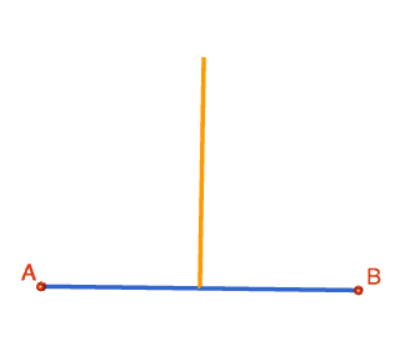# Constructing the Perpendicular Bisector of a Straight Line

Compass and straight edge constructions are of interest to mathematicians, not only in the field of geometry, but also in algebra. For thousands of years, beginning with the Ancient Babylonians, mathematicians were interested in the problem of "squaring the circle" (drawing a square with the same area as a circle) using a straight edge and compass. This problem is equivalent to finding the area of a circle. It turns out that this is impossible, but no-one managed to prove this until 1882!

However, constructing the perpendicular bisector of a straight line segment (a line perpendicular to the line segment that cuts it in half) is possible. For this construction, you will need a straight edge (ruler - but you won't be measuring anything), pair of compasses, a pencil and paper. I have drawn the pictures using the robocompass app. It's fun to play with, and you can use it to do all sorts of geometric constructions. There's a little bit of coding to learn, but a list of instructions is provided. Once you've written your little program, you can get out the popcorn, sit back and watch the construction. Be warned that, when you are using it to draw arcs, the robocompass compass point may appear to be a little away from the centre, but the drawing is actually accurate.

## The ConstructionStep 1: Start out by drawing the line segment $AB$ that you want to bisect. Please don't be like Sam! Use a ruler to draw this.Step 2: Place the tip of your pair of compasses on point $A$, and open them out to about $\dfrac{2}{3}$ of the way along your line segment. You are going to draw four arcs of this radius, so make sure you keep it fixed. Draw two arcs (one above and one below the line segment). The picture shows the tip of the compass a little away from point $A$. This is just a problem with the animation. The arcs are actually centred at point $A$, so make sure your compass tip is exactly on point $A$.Step 3: Keeping your compasses at the same radius as in the preceding step, place the tip of your pair of compasses on point $B$. Draw two arcs (one above and one below the line segment). The picture shows the tip of the compass a little away from point $B$. This is just a problem with the animation. The arcs are actually centred at point $B$, so make sure your compass tip is exactly on point $B$. As shown in the picture, your arcs need to intersect the arcs drawn in step 2.Step 4: Use your ruler to join the points of intersection of both pairs of arcs (above and below the line).This is the finished product. You can rub the arcs out, if you like. The vertical line (shown in orange) is at right angles to line segment $AB$, and cuts it in half. Now you can do some measuring and use your ruler to check!### Description

This tutorial will expose you to Rulers and how to use them. We will also have a close look as set square and compass constructions. Your feedback is important to us, if you like any other topic covered under this tutorial, please do let us know.

### Audience

Year 10 or higher

### Learning Objectives

Ruler and compass constructions and more

Author: Subject Coach
Added on: 27th Sep 2018

You must be logged in as Student to ask a Question.

None just yet!